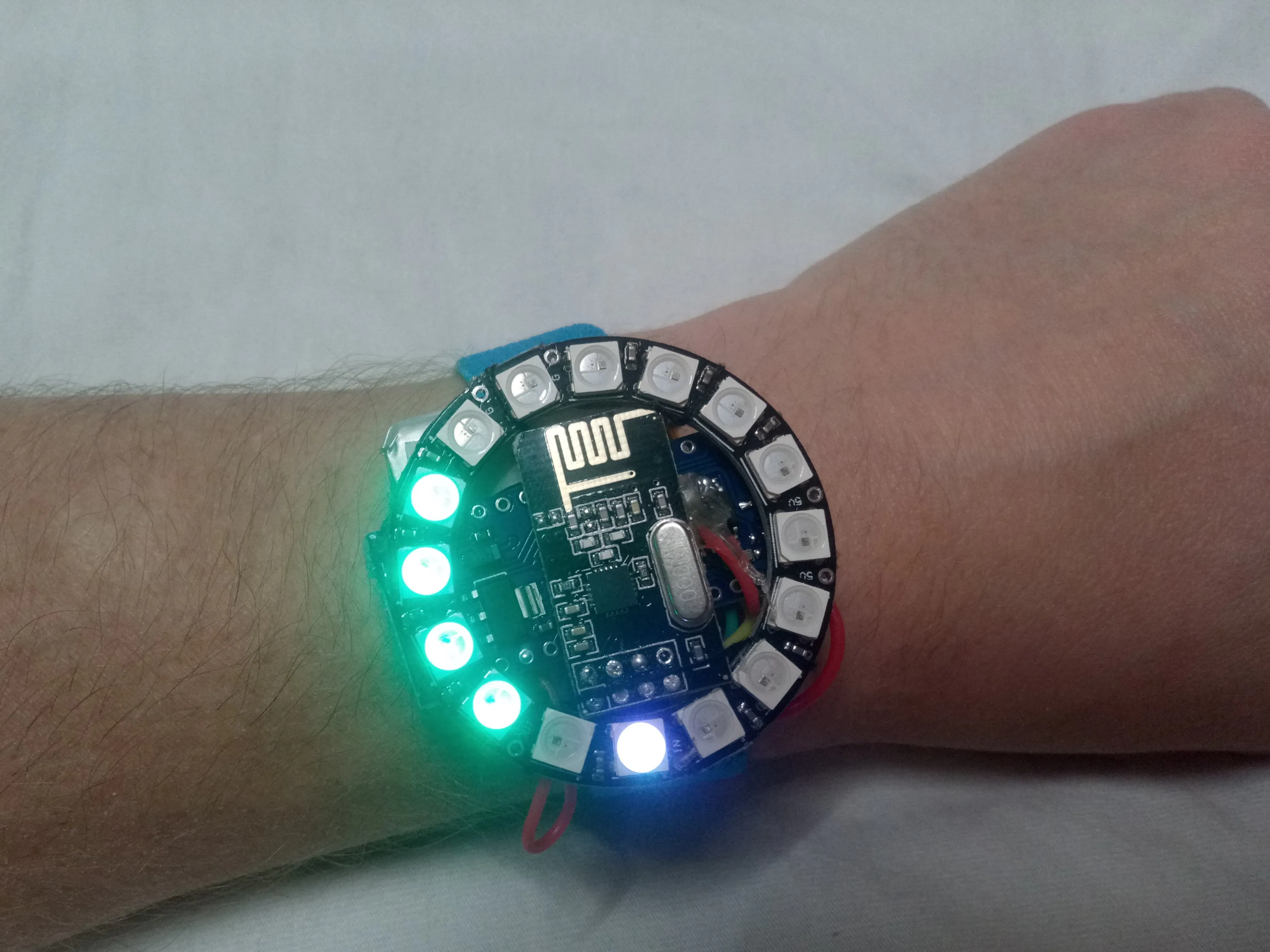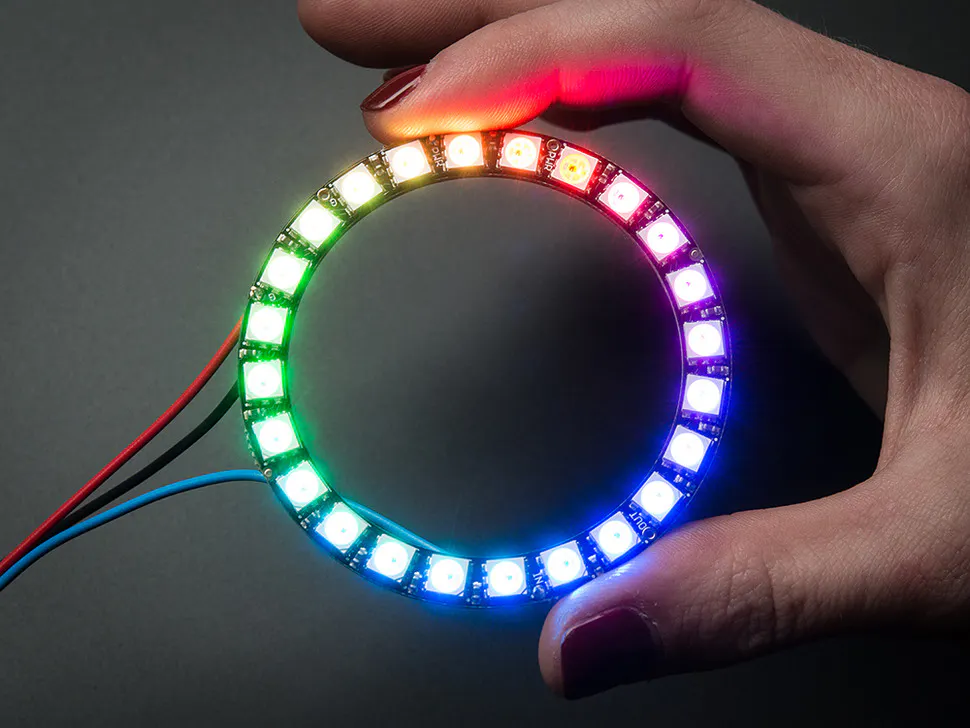Heart Rate Monitor (Wearable and Wireless Using ECG)

A rather convenient device to monitor heart rate while running.

IntermediateFull instructions provided6 hours43,078Things used in this project

Hardware components

 uECG device For actually measuring BPM. It can send data via nRF24-compatible protocol
×1Arduino Nano R3
×1
 nRF24 Module (Generic) Any module would work here. Required to receive BPM data from uECG
×1Adafruit NeoPixel Ring: WS2812 5050 RGB LED
×1
 LiPo battery Any battery with 200+ mAh capacity
×1

Hand tools and fabrication machinesSoldering iron (generic)

Code

bpm_watch.ino

Arduino
#ifdef __AVR__
#include <avr/power.h>
#endif

#include <SPI.h>
#include <RF24.h>
#include <RF24_config.h>
#include <nRF24L01.h>

int rf_cen = 9; //nRF24 chip enable pin
int rf_cs = 8; //nRF24 CS pin

RF24 rf(rf_cen, rf_cs);
//pipe address - hardcoded on uECG side
uint8_t pipe_rx = {0x0E, 0xE6, 0x0D, 0xA7, 0, 0, 0, 0};

// Which pin on the Arduino is connected to the NeoPixels?
#define PIN            5
// How many NeoPixels are attached to the Arduino?
#define NUMPIXELS      16

uint8_t  swapbits(uint8_t a){ //uECG pipe address uses swapped bits order
// reverse the bit order in a single byte
uint8_t v = 0;
if(a & 0x80) v |= 0x01;
if(a & 0x40) v |= 0x02;
if(a & 0x20) v |= 0x04;
if(a & 0x10) v |= 0x08;
if(a & 0x08) v |= 0x10;
if(a & 0x04) v |= 0x20;
if(a & 0x02) v |= 0x40;
if(a & 0x01) v |= 0x80;
return v;
}

void setup() {
pixels.begin(); // This initializes the NeoPixel library.

for(int i=0;i<NUMPIXELS;i++){
pixels.setPixelColor(i, pixels.Color(1,1,1));
}
pixels.show();

//nRF24 requires relatively slow SPI, probably would work at 2MHz too
SPI.begin();
SPI.setBitOrder(MSBFIRST);
SPI.beginTransaction(SPISettings(1000000, MSBFIRST, SPI_MODE0));

for(int x = 0; x < 8; x++) //nRF24 and uECG have different bit order for pipe address
pipe_rx[x] = swapbits(pipe_rx[x]);

rf.begin();
rf.setDataRate(RF24_1MBPS);
rf.setChannel(22);
rf.setRetries(0, 0);
rf.setAutoAck(0);
rf.setCRCLength(RF24_CRC_DISABLED);
rf.disableCRC();
rf.startListening(); //listen for uECG data
//Note that uECG should be switched into raw data mode (via long button press)
//in order to send compatible packets, by default it sends data in BLE mode
//which cannot be received by nRF24
}

long last_pix_upd = 0;
byte in_pack;
int rf_bpm = 0;
int bpm_hist; //since we disabled CRC, need to filter incoming data

void loop()
{
if(rf.available())
{
int bb = in_pack; //BPM is located at the 5th byte of the packet
//detailed packet structure is in uECG docs

//since we have no CRC for compatibility reasons, we need to filter
//incoming data, radio channel could be noisy. We compare last 5
//received BPM values and use one only if all 5 were the same.
//Since uECG sends about 100 packets per second, it won't cause
//any noticeable delay in displaying data
for(int n = 0; n < 5-1; n++) //shift bpm history array by 1
bpm_hist[n] = bpm_hist[n+1];
bpm_hist = bb; //add new bpm value
for(int n = 0; n < 5; n++) //check if all are equal
if(bpm_hist[n] != bb) bb = -1;
if(bb > 0) //if yes - store it as new received BPM
rf_bpm = bb;
}
long ms = millis();
if(ms - last_pix_upd > 10) //don't update pixels too often
{
int r, g, b;
last_pix_upd = ms;
int bpm = rf_bpm;
int max_bright = 160; //value of maximum brightness, max 255. But you don't always want it at max :)
float dd = 25; //change in BPM between color tones (blue->green->yellow->pink->red)
float t1 = 90, t2, t3, t4; //t1 - "base" BPM, lower than t1 would be blue
t2 = t1 + dd;
t3 = t2 + dd;
t4 = t3 + dd;
//code for changing color depending in which t1...t4 range we are now
if(bpm < t1){ r = 0; g = 0; b = max_bright; }
else if(bpm < t2) { r = 0; g = max_bright * (bpm-t1)/dd; b = max_bright - g; }
else if(bpm < t3) { r = max_bright * (bpm-t2)/dd; g = max_bright - r; b = r/4; }
else if(bpm < t4) { r = max_bright; g = 0; b = max_bright/2 - max_bright * (bpm-t3)/(2*dd); }
else {r = max_bright; g = 0; b = 0; }
int on_pixels = (bpm-80)/8; //since it's intended for running, I'm not
//showing anything less than 80 BPM, this way it's more sensitive in
for(int i=0;i<NUMPIXELS;i++)
{
//pixels are set from last to first for no particular reason, would
//work just as fine if set from first to last
if(i < on_pixels) pixels.setPixelColor(NUMPIXELS-i-1, pixels.Color(r,g,b));
else pixels.setPixelColor(NUMPIXELS-i-1, pixels.Color(0,0,0)); //turn off all other LEDs
}
pixels.show();
}
}

Credits

Dmitry Dziuba

5 projects • 62 followers
I'm an electronics engineer with background in AI and physics